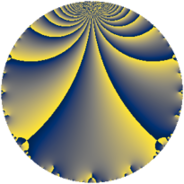# Properties

 Label 2151.2.a.bLevel $2151$ Weight $2$ Character orbit 2151.a Self dual yes Analytic conductor $17.176$ Analytic rank $1$ Dimension $2$ CM no Inner twists $1$

# Related objects

## Newspace parameters

 Level: $$N$$ $$=$$ $$2151 = 3^{2} \cdot 239$$ Weight: $$k$$ $$=$$ $$2$$ Character orbit: $$[\chi]$$ $$=$$ 2151.a (trivial)

## Newform invariants

 Self dual: yes Analytic conductor: $$17.1758214748$$ Analytic rank: $$1$$ Dimension: $$2$$ Coefficient field: $$\Q(\sqrt{5})$$ Defining polynomial: $$x^{2} - x - 1$$ Coefficient ring: $$\Z[a_1, a_2]$$ Coefficient ring index: $$1$$ Twist minimal: no (minimal twist has level 717) Fricke sign: $$1$$ Sato-Tate group: $\mathrm{SU}(2)$

## $q$-expansion

Coefficients of the $$q$$-expansion are expressed in terms of $$\beta = \frac{1}{2}(1 + \sqrt{5})$$. We also show the integral $$q$$-expansion of the trace form.

 $$f(q)$$ $$=$$ $$q -\beta q^{2} + ( -1 + \beta ) q^{4} -3 q^{5} + ( 2 - 2 \beta ) q^{7} + ( -1 + 2 \beta ) q^{8} +O(q^{10})$$ $$q -\beta q^{2} + ( -1 + \beta ) q^{4} -3 q^{5} + ( 2 - 2 \beta ) q^{7} + ( -1 + 2 \beta ) q^{8} + 3 \beta q^{10} + ( 1 - 2 \beta ) q^{11} + 2 q^{14} -3 \beta q^{16} -3 q^{17} + ( 2 + 4 \beta ) q^{19} + ( 3 - 3 \beta ) q^{20} + ( 2 + \beta ) q^{22} + ( 2 + 2 \beta ) q^{23} + 4 q^{25} + ( -4 + 2 \beta ) q^{28} + 3 q^{29} + ( -1 - 2 \beta ) q^{31} + ( 5 - \beta ) q^{32} + 3 \beta q^{34} + ( -6 + 6 \beta ) q^{35} + ( -8 + 2 \beta ) q^{37} + ( -4 - 6 \beta ) q^{38} + ( 3 - 6 \beta ) q^{40} + 4 \beta q^{41} + 6 q^{43} + ( -3 + \beta ) q^{44} + ( -2 - 4 \beta ) q^{46} + ( -6 + 4 \beta ) q^{47} + ( 1 - 4 \beta ) q^{49} -4 \beta q^{50} + 8 \beta q^{53} + ( -3 + 6 \beta ) q^{55} + ( -6 + 2 \beta ) q^{56} -3 \beta q^{58} + ( -10 + 2 \beta ) q^{59} + q^{61} + ( 2 + 3 \beta ) q^{62} + ( 1 + 2 \beta ) q^{64} + ( -5 - 6 \beta ) q^{67} + ( 3 - 3 \beta ) q^{68} -6 q^{70} + ( -4 + 4 \beta ) q^{71} + ( -2 + 8 \beta ) q^{73} + ( -2 + 6 \beta ) q^{74} + ( 2 + 2 \beta ) q^{76} + ( 6 - 2 \beta ) q^{77} -6 q^{79} + 9 \beta q^{80} + ( -4 - 4 \beta ) q^{82} + ( -5 - 2 \beta ) q^{83} + 9 q^{85} -6 \beta q^{86} -5 q^{88} + 2 \beta q^{92} + ( -4 + 2 \beta ) q^{94} + ( -6 - 12 \beta ) q^{95} + ( -6 + 6 \beta ) q^{97} + ( 4 + 3 \beta ) q^{98} +O(q^{100})$$ $$\operatorname{Tr}(f)(q)$$ $$=$$ $$2q - q^{2} - q^{4} - 6q^{5} + 2q^{7} + O(q^{10})$$ $$2q - q^{2} - q^{4} - 6q^{5} + 2q^{7} + 3q^{10} + 4q^{14} - 3q^{16} - 6q^{17} + 8q^{19} + 3q^{20} + 5q^{22} + 6q^{23} + 8q^{25} - 6q^{28} + 6q^{29} - 4q^{31} + 9q^{32} + 3q^{34} - 6q^{35} - 14q^{37} - 14q^{38} + 4q^{41} + 12q^{43} - 5q^{44} - 8q^{46} - 8q^{47} - 2q^{49} - 4q^{50} + 8q^{53} - 10q^{56} - 3q^{58} - 18q^{59} + 2q^{61} + 7q^{62} + 4q^{64} - 16q^{67} + 3q^{68} - 12q^{70} - 4q^{71} + 4q^{73} + 2q^{74} + 6q^{76} + 10q^{77} - 12q^{79} + 9q^{80} - 12q^{82} - 12q^{83} + 18q^{85} - 6q^{86} - 10q^{88} + 2q^{92} - 6q^{94} - 24q^{95} - 6q^{97} + 11q^{98} + O(q^{100})$$

## Embeddings

For each embedding $$\iota_m$$ of the coefficient field, the values $$\iota_m(a_n)$$ are shown below.

For more information on an embedded modular form you can click on its label.

Label $$\iota_m(\nu)$$ $$a_{2}$$ $$a_{3}$$ $$a_{4}$$ $$a_{5}$$ $$a_{6}$$ $$a_{7}$$ $$a_{8}$$ $$a_{9}$$ $$a_{10}$$
1.1
 1.61803 −0.618034
−1.61803 0 0.618034 −3.00000 0 −1.23607 2.23607 0 4.85410
1.2 0.618034 0 −1.61803 −3.00000 0 3.23607 −2.23607 0 −1.85410
 $$n$$: e.g. 2-40 or 990-1000 Significant digits: Format: Complex embeddings Normalized embeddings Satake parameters Satake angles

## Atkin-Lehner signs

$$p$$ Sign
$$3$$ $$-1$$
$$239$$ $$-1$$

## Inner twists

This newform does not admit any (nontrivial) inner twists.

## Twists

By twisting character orbit
Char Parity Ord Mult Type Twist Min Dim
1.a even 1 1 trivial 2151.2.a.b 2
3.b odd 2 1 717.2.a.b 2

By twisted newform orbit
Twist Min Dim Char Parity Ord Mult Type
717.2.a.b 2 3.b odd 2 1
2151.2.a.b 2 1.a even 1 1 trivial

## Hecke kernels

This newform subspace can be constructed as the intersection of the kernels of the following linear operators acting on $$S_{2}^{\mathrm{new}}(\Gamma_0(2151))$$:

 $$T_{2}^{2} + T_{2} - 1$$ $$T_{5} + 3$$

## Hecke characteristic polynomials

$p$ $F_p(T)$
$2$ $$-1 + T + T^{2}$$
$3$ $$T^{2}$$
$5$ $$( 3 + T )^{2}$$
$7$ $$-4 - 2 T + T^{2}$$
$11$ $$-5 + T^{2}$$
$13$ $$T^{2}$$
$17$ $$( 3 + T )^{2}$$
$19$ $$-4 - 8 T + T^{2}$$
$23$ $$4 - 6 T + T^{2}$$
$29$ $$( -3 + T )^{2}$$
$31$ $$-1 + 4 T + T^{2}$$
$37$ $$44 + 14 T + T^{2}$$
$41$ $$-16 - 4 T + T^{2}$$
$43$ $$( -6 + T )^{2}$$
$47$ $$-4 + 8 T + T^{2}$$
$53$ $$-64 - 8 T + T^{2}$$
$59$ $$76 + 18 T + T^{2}$$
$61$ $$( -1 + T )^{2}$$
$67$ $$19 + 16 T + T^{2}$$
$71$ $$-16 + 4 T + T^{2}$$
$73$ $$-76 - 4 T + T^{2}$$
$79$ $$( 6 + T )^{2}$$
$83$ $$31 + 12 T + T^{2}$$
$89$ $$T^{2}$$
$97$ $$-36 + 6 T + T^{2}$$